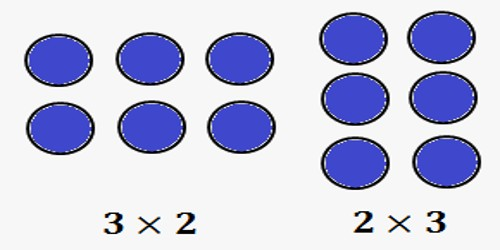Mathematic

# Commutative Property of Multiplication of Two Complex NumbersA complex number is a number of the form a + bi, where ‘a’ and ‘b’ are real numbers and ‘I’ is an indeterminate satisfying i2 = −1. For example, 2 + 3i is a complex number.

The equations x2 + 5 = 0, x2 + 10 = 0, x2 = -1 are not solvable in the real number system i.e, these equations has no real roots. For example, i is the solution of the equation x2 = -1 and it has two solutions i.e., x = ± i, where √-1.

The number, ‘I’ is called an imaginary number. Generally, the square root of any negative real number is called imaginary number. The concept of imaginary numbers was first introduced by mathematician “Euler”. He was the one who introduced ‘i’ (read as ‘iota’) to represent √-1. He also defined i2 = -1.

Multiplication of Two Complex Numbers

Multiplication of two complex numbers is also a complex number.

In other words, the product of two complex numbers can be expressed in the standard form A + iB where A and B are real.

Let z1 = p + iq and z2 = r + is be two complex numbers (p, q, r and s are real), then their product z1z2 is defined as

z1z2 = (pr – qs) + i(ps + qr).

Commutative property of multiplication of two complex numbers:

For any two complex number z1 and z2, we have z1z2 = z2z1.

Proof:

Let z1 = p + iq and z2 = r + is, where p, q, r and s are real numbers. Them

z1z2 = (p + iq)(r + is) = (pr – qs) + i(ps – rq)

and z2z1 = (r + is) (p + iq) = (rp – sq) + i(sp – qr)

= (pr – qs) + i(ps – rq), [Using the commutative of multiplication of real numbers]

Therefore, z1z2 = z2z1

Thus, z1z2 = z2z1 for all z1, z2 ϵ C.

Hence, the multiplication of complex numbers is commutative on C.

Examples of commutative property of multiplication of two complex numbers:

1. Show that multiplication of two complex numbers (2 + 3i) and (3 + 4i) is commutative.

Solution:

Let, z1 = (2 + 3i) and z2 = (3 + 4i)

Now, z1z2 = (2 + 3i)(3 + 4i)

= (2 ∙ 3 – 3 ∙ 4) + (2 ∙ 4 + 3 ∙ 3)i

= (6 – 12) + (8 + 9)i

= – 6 + 17i

Again, z2z1= (3 + 4i)(2 + 3i)

= (3 ∙ 2 – 4 ∙ 3) + (3 ∙ 3 + 2 ∙ 4)i

= (6 – 12) + (9 + 8)i

= -6 + 17i

Therefore, z1z2 = z2z1

Thus, z1z2 = z2z1 for all z1, z2 ϵ C.

Hence, the multiplication of two complex numbers (2 + 3i) and (3 + 4i) is commutative.

1. Show that multiplication of two complex numbers (3 – 2i) and (-5 + 4i) is commutative.

Solution:

Let, z1 = (3 – 2i) and z2 = (-5 + 4i)

Now, z1z2 = (3 – 2i)(-5 + 4i)

= (3 ∙ (-5) – (-2) ∙ 4) + ((-2) ∙ 4 + (-5) ∙ (-2))i

= (-15 – (-8)) + ((-8) + 10)i

= (-15 + 8) + (-8 + 10)i = – 7 + 2i

Again, z2z1 = (-5 + 4i)(3 – 2i)

= ((-5) ∙ 3 – 4 ∙ (-2)) + (4 ∙ 3 + (-2) ∙ 4)i

= (-15 + 8) + (12 – 8)i = -7 + 2i

Therefore, z1z2 = z2z1

Thus, z1z2 = z2z1 for all z1, z2 ϵ C.

Hence, the multiplication of two complex numbers (3 – 2i) and (-5 + 4i) is commutative.

Information Source: